ggplot {ggbio} R Documentation

## ggplot generic method

### Description

Usage is similar to `ggplot` function in ggplot2 package, but support more objects defined in Bioconductor, or even for object like matrix, which intitialize a ggbio object. It can be used to declare the input data frame for a graphic and to specify the set of plot aesthetics intended to be common throughout all subsequent layers unless specifically overridden. And more than that, it store the original data object before coercion into the returned object. Please check the behavior for `mold` method to see how each object coerced into data.frame.

### Usage

```## S4 method for signature 'GRanges'
ggplot(data, ...)
## S4 method for signature 'GRangesList'
ggplot(data, ...)
## S4 method for signature 'IRanges'
ggplot(data, ...)
## S4 method for signature 'Seqinfo'
ggplot(data, ...)
## S4 method for signature 'matrix'
ggplot(data, ...)
## S4 method for signature 'Views'
ggplot(data, ...)
## S4 method for signature 'ExpressionSet'
ggplot(data, ...)
## S4 method for signature 'SummarizedExperiment'
ggplot(data, assay.id = 1, ...)
## S4 method for signature 'VCF'
ggplot(data, ...)
## S4 method for signature 'GappedAlignments'
ggplot(data, ...)
## S4 method for signature 'BamFile'
ggplot(data, ...)
## S4 method for signature 'character'
ggplot(data, ...)
## S4 method for signature 'TranscriptDb'
ggplot(data, ...)
## S4 method for signature 'BSgenome'
ggplot(data, ...)
## S4 method for signature 'Rle'
ggplot(data, ...)
## S4 method for signature 'RleList'
ggplot(data, ...)
```

### Arguments

 `data` original data object. `...` other arguments passed to specific methods. `assay.id` index of assay you are using when multiple assays exist.

### Details

The biggest difference for objects returned by `ggplot` in ggbio from ggplot2, is we always keep the original data copy, this is useful because in ggbio, our starting point is not always data.frame, many special statistical transformation is computed upon original data objects instead of coerced data.frame. This is a hack to follow ggplot2's API while allow our own defined components to trace back to original data copy and do the transformation. For objects supported by `mold` we transform them to data.frame stored along the original data set, for objects which not supported by `mold` method, we only store the original copy for ggbio specific graphics.

`ggplot()` is typically used to construct a plot incrementally, using the `+` operator to add layers to the existing ggplot object. This is advantageous in that the code is explicit about which layers are added and the order in which they are added. For complex graphics with multiple layers, initialization with `ggplot` is recommended. You can always call `qplot` in package ggplot2 or `autoplot` in ggbio for convenient usage.

There are three common ways to invoke `ggplot`:

• `ggplot(df, aes(x, y, <other aesthetics>))`

• `ggplot(df)`

• `ggplot()`

The first method is recommended if all layers use the same data and the same set of aesthetics, although this method can also be used to add a layer using data from another data frame. The second method specifies the default data frame to use for the plot, but no aesthetics are defined up front. This is useful when one data frame is used predominantly as layers are added, but the aesthetics may vary from one layer to another. The third method initializes a skeleton `ggplot` object which is fleshed out as layers are added. This method is useful when multiple data frames are used to produce different layers, as is often the case in complex graphics.

The examples below illustrate how these methods of invoking `ggplot` can be used in constructing a graphic.

### Value

a return `ggbio` object, which is a subclass of `ggplot` defined in ggplot2 package, but that's more, a '.data' list entry is stored with the returned object.

### Author(s)

Tengfei Yin

`mold`, `ggbio`

### Examples

``````set.seed(1)
N <- 100
library(GenomicRanges)
## GRanges
gr <- GRanges(seqnames =
sample(c("chr1", "chr2", "chr3"),
size = N, replace = TRUE),
IRanges(
start = sample(1:300, size = N, replace = TRUE),
width = sample(70:75, size = N,replace = TRUE)),
strand = sample(c("+", "-", "*"), size = N,
replace = TRUE),
value = rnorm(N, 10, 3), score = rnorm(N, 100, 30),
sample = sample(c("Normal", "Tumor"),
size = N, replace = TRUE),
pair = sample(letters, size = N,
replace = TRUE))
## automatically facetting and assign y
## this must mean geom_rect support GRanges object
ggplot(gr) + geom_rect()
``````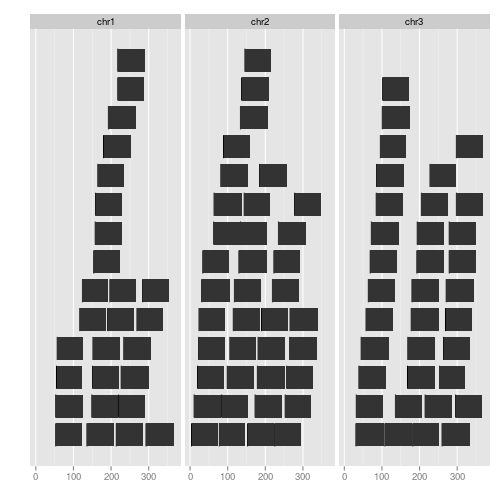``````ggplot(gr) + geom_alignment()
``````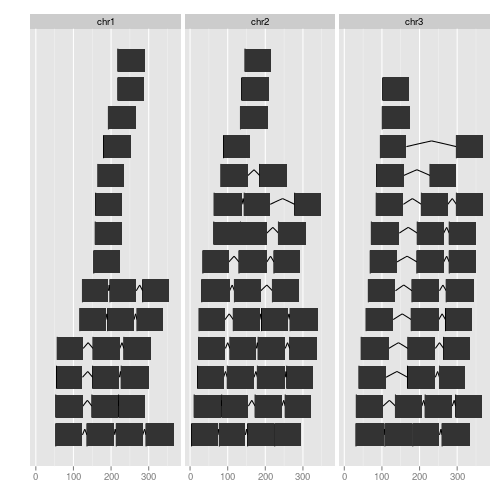``````ggplot() + geom_alignment(gr)
````````````## use pure ggplot2's geom_rect, no auto facet
ggplot(gr) + ggplot2::geom_rect(aes(xmin = start, ymin = score,
xmax = end, ymax = score + 1))
``````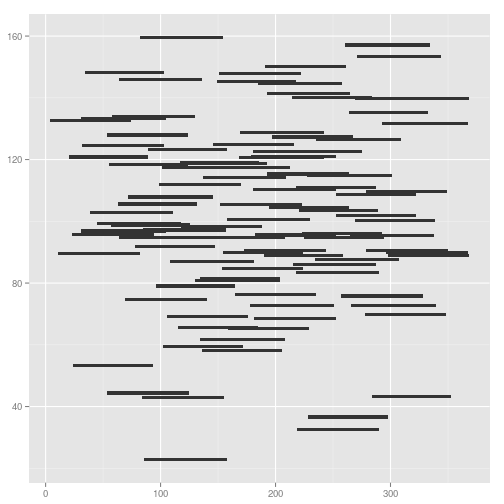``````## GRangesList
grl <- split(gr, values(gr)\$pair)
ggplot(grl) + geom_alignment()
````````````ggplot(grl) + geom_rect()
````````````ggplot(grl) + ggplot2::geom_rect(aes(xmin = start, ymin = score,
xmax = end, ymax = score + 1))
````````````## IRanges
ir <- ranges(gr)
ggplot(ir) + geom_rect()
``````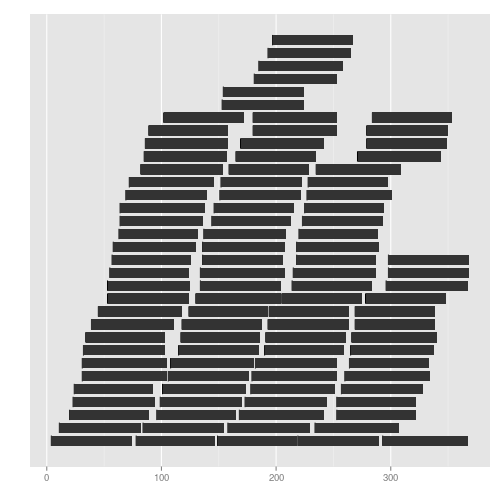``````ggplot(ir) + layout_circle(geom = "rect")
````````````## Seqinfo
seqlengths(gr) <- c(400, 500, 420)
ggplot(seqinfo(gr)) + geom_point(aes(x = midpoint, y = seqlengths))
``````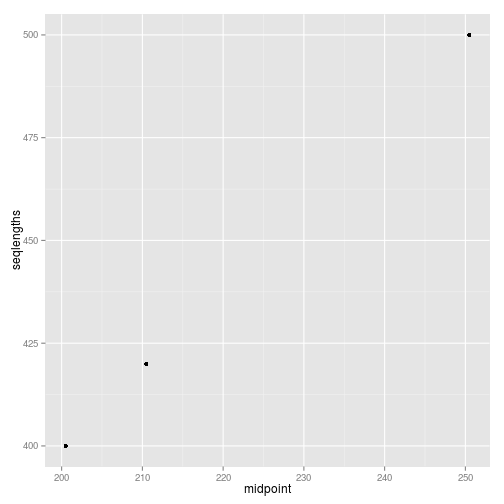``````## matrix
mx <- matrix(1:12, nrow = 3)
ggplot(mx, aes(x = x, y = y)) + geom_raster(aes(fill = value))
``````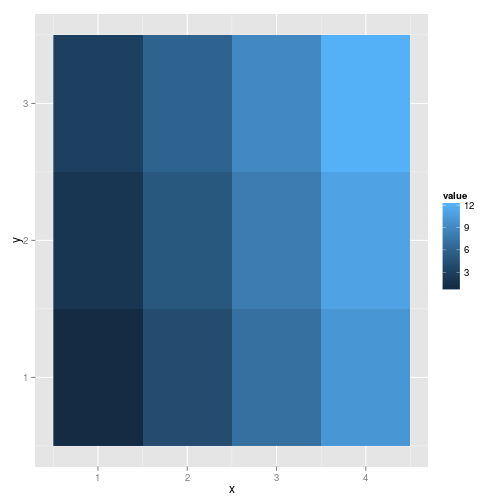``````## row is the factor
ggplot(mx, aes(x = x, y = row)) + geom_raster(aes(fill = value))
``````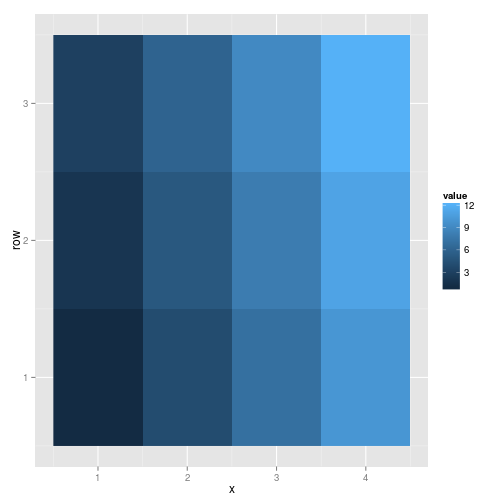``````colnames(mx)
``````
``````## NULL
``````
``````colnames(mx) <- letters[1:ncol(mx)]
mx
``````
``````##      a b c  d
## [1,] 1 4 7 10
## [2,] 2 5 8 11
## [3,] 3 6 9 12
``````
``````## has extra 'colnames'
ggplot(mx, aes(x = x, y = row)) + geom_raster(aes(fill = colnames))
``````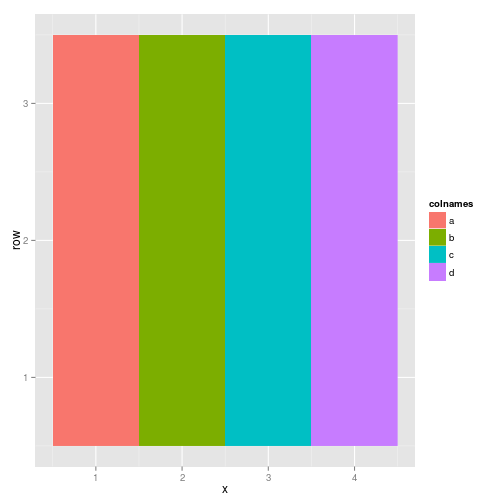``````rownames(mx)
``````
``````## NULL
``````
``````rownames(mx) <- LETTERS[1:nrow(mx)]
ggplot(mx, aes(x = x, y = row)) + geom_raster(aes(fill = rownames))
``````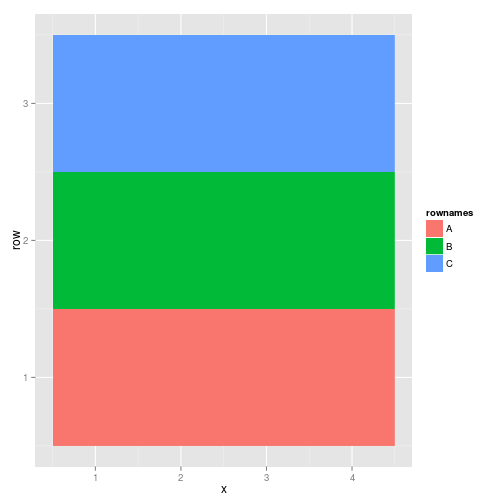``````## please check autoplot, matrix for more control
##  Views
## ExpressionSet
library(Biobase)
data(sample.ExpressionSet)
sample.ExpressionSet
``````
``````## ExpressionSet (storageMode: lockedEnvironment)
## assayData: 500 features, 26 samples
##   element names: exprs, se.exprs
## protocolData: none
## phenoData
##   sampleNames: A B ... Z (26 total)
##   varLabels: sex type score
## featureData: none
## experimentData: use 'experimentData(object)'
## Annotation: hgu95av2
``````
``````set.seed(1)
## select 50 features
idx <- sample(seq_len(dim(sample.ExpressionSet)), size = 50)
eset <- sample.ExpressionSet[idx,]
ggplot(eset) + geom_tile(aes(x = x, y = y, fill = value))
``````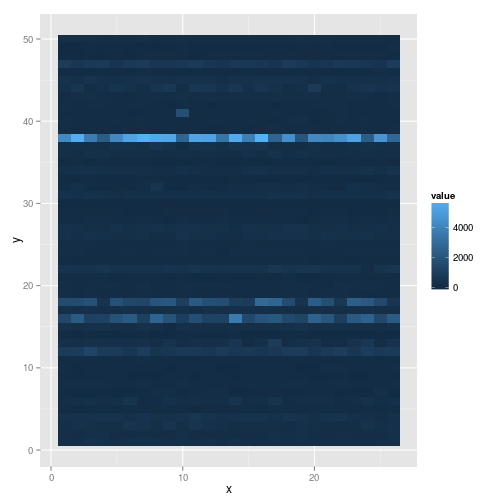``````## please check autoplot,matrix method which gives you more control
``````
``````##   x y value row col colnames rownames    sex    type score
## A 1 1 177.1   1   1        A 31372_at Female Control  0.75
## B 2 1 171.2   1   2        B 31372_at   Male    Case  0.40
## C 3 1 277.2   1   3        C 31372_at   Male Control  0.73
## D 4 1 190.2   1   4        D 31372_at   Male    Case  0.42
## E 5 1 138.4   1   5        E 31372_at Female    Case  0.93
## F 6 1 174.0   1   6        F 31372_at   Male Control  0.22
``````
``````ggplot(eset) + geom_tile(aes(x = x, y = y, fill = sex))
``````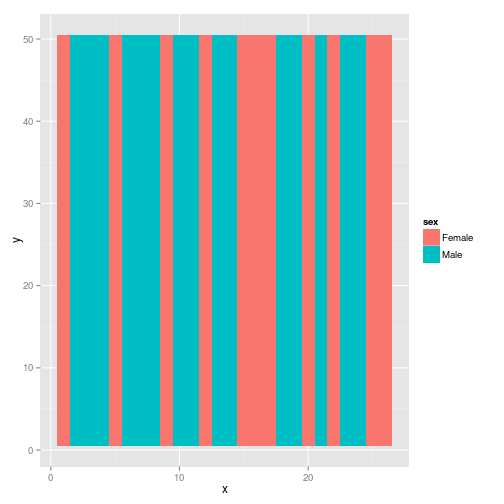``````ggplot(eset) + geom_tile(aes(x = x, y = y, fill = type))
``````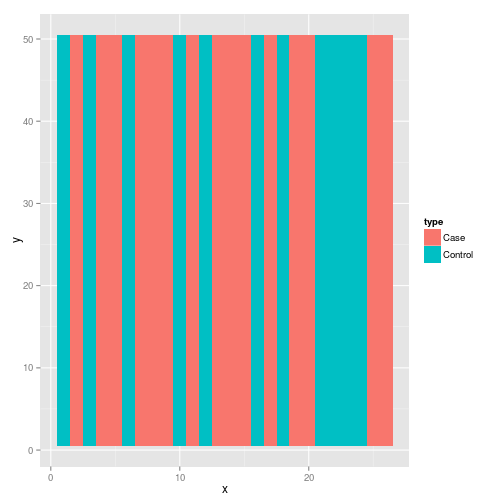``````## Rle
library(IRanges)
lambda <- c(rep(0.001, 4500), seq(0.001, 10, length = 500),
seq(10, 0.001, length = 500))
xVector <- rpois(1e4, lambda)
xRle <- Rle(xVector)
ggplot(xRle) + geom_tile(aes(x = x, y = y, fill = value))
``````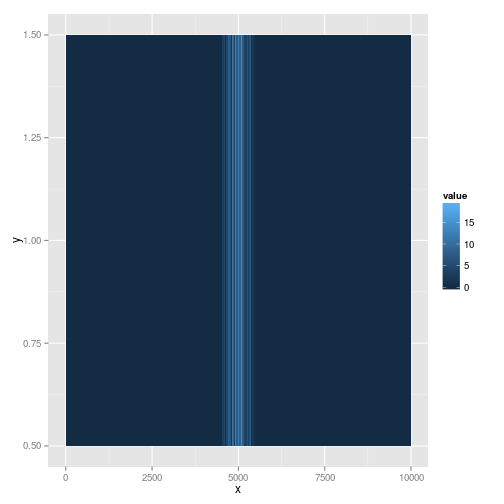``````## RleList
xRleList <- RleList(xRle, 2L * xRle)
xRleList
``````
``````## SimpleRleList of length 2
## []
## numeric-Rle of length 10000 with 829 runs
##   Lengths:  729    1  208    1 1599    1 ...    1    1    1    5    1 4512
##   Values :    0    1    0    1    0    1 ...    1    0    1    0    1    0
##
## []
## numeric-Rle of length 10000 with 829 runs
##   Lengths:  729    1  208    1 1599    1 ...    1    1    1    5    1 4512
##   Values :    0    2    0    2    0    2 ...    2    0    2    0    2    0
``````
``````ggplot(xRleList) + geom_tile(aes(x = x, y = y, fill = value)) +
facet_grid(group~.)
``````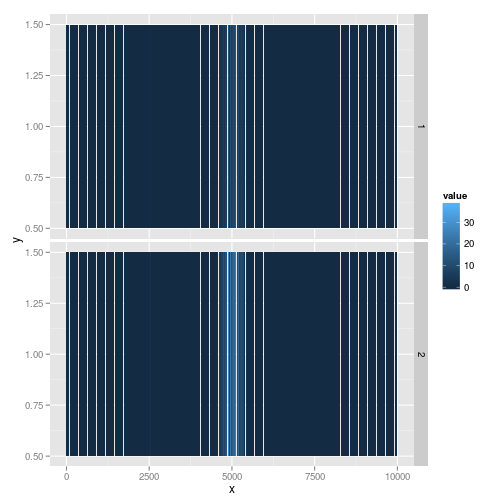``````names(xRleList) <- c("a" ,"b")
ggplot(xRleList) + geom_tile(aes(x = x, y = y, fill = value)) +
facet_grid(group~.)
``````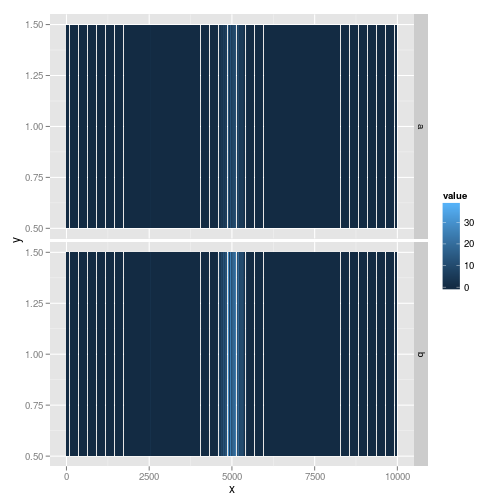``````## SummarizedExperiments
library(GenomicRanges)
nrows <- 200; ncols <- 6
counts <- matrix(runif(nrows * ncols, 1, 1e4), nrows)
counts2 <- matrix(runif(nrows * ncols, 1, 1e4), nrows)
rowData <- GRanges(rep(c("chr1", "chr2"), c(50, 150)),
IRanges(floor(runif(200, 1e5, 1e6)), width=100),
strand=sample(c("+", "-"), 200, TRUE))
colData <- DataFrame(Treatment=rep(c("ChIP", "Input"), 3),
row.names=LETTERS[1:6])
sset <- SummarizedExperiment(assays=SimpleList(counts=counts,
counts2 = counts2),
rowData=rowData, colData=colData)
ggplot(sset) + geom_raster(aes(x = x, y = y , fill = value))
``````
``````## Assay index: 1 used
``````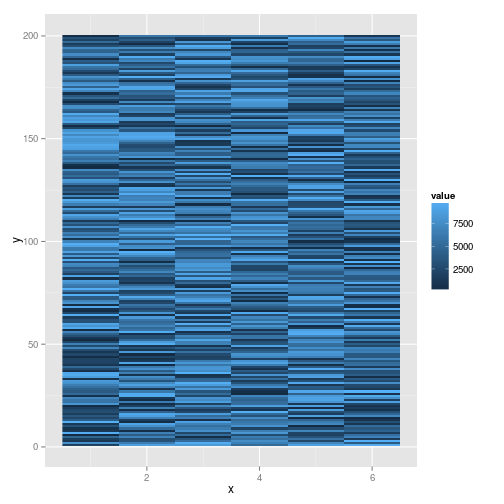[Package ggbio version 1.5.20 ]